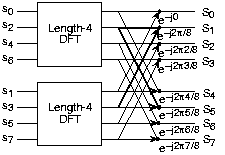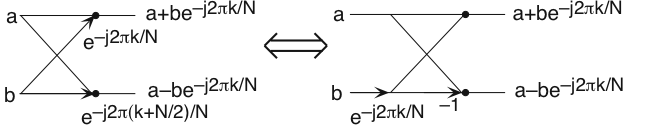0.3 Fast fourier transform (fft)  (Page 2/2)

Now for the fun. Because $N=2^{L}$ , each of the half-length transforms can be reduced to two quarter-length transforms, each of these to twoeighth-length ones, etc. This decomposition continues until we are left with length-2 transforms. This transform is quitesimple, involving only additions. Thus, the first stage of the FFT has $\frac{N}{2}$ length-2 transforms (see the bottom part of [link] ). Pairs of these transforms are combined by adding one to the other multiplied by a complexexponential. Each pair requires 4 additions and 2 multiplications, giving a total number of computations equaling $6·\frac{N}{4}=\frac{3N}{2}$ . This number of computations does not change from stage to stage.Because the number of stages, the number of times the length can be divided by two, equals $\log_{2}N$ , the number of arithmetic operations equals $\frac{3N}{2}\log_{2}N$ , which makes the complexity of the FFT $O(N\log_{2}N)$ .The initial decomposition of a length-8 DFT into the terms using even- and odd-indexed inputs marks the first phase ofdeveloping the FFT algorithm. When these half-length transforms are successively decomposed, we are left with the diagram shownin the bottom panel that depicts the length-8 FFT computation.

Doing an example will make computational savings more obvious. Let's look at the detailsof a length-8 DFT. As shown on [link] , we first decompose the DFT into two length-4 DFTs, with the outputs added and subtracted together in pairs.Considering [link] as the frequency index goes from 0 through 7, we recycle values fromthe length-4 DFTs into the final calculation because of the periodicity of the DFT output. Examining how pairs of outputsare collected together, we create the basic computational element known as a butterfly ( [link] ).The basic computational element of the fast Fourier transform is the butterfly. It takes two complex numbers, representedby a and b , and forms the quantities shown. Each butterfly requires onecomplex multiplication and two complex additions. By considering together the computations involving common output frequencies from the two half-length DFTs, we see that the twocomplex multiplies are related to each other, and we can reduce our computational work even further. By further decomposing thelength-4 DFTs into two length-2 DFTs and combining their outputs, we arrive at the diagram summarizing the length-8 fastFourier transform ( [link] ). Although most of the complex multiplies are quite simple(multiplying by $e^{-(i\frac{\pi }{2})}$ means swapping real and imaginary parts and changing their signs), let's count those forpurposes of evaluating the complexity as full complex multiplies. We have $\frac{N}{2}=4$ complex multiplies and $N=8$ complex additions for each stage and $\log_{2}N=3$ stages, making the number of basic computations $\frac{3N}{2}\log_{2}N$ as predicted.

Note that the ordering of the input sequence in the two parts of [link] aren't quite the same. Why not? How is the ordering determined?

The upper panel has not used the FFT algorithm to compute the length-4 DFTs while the lower one has. The ordering isdetermined by the algorithm.

Other "fast" algorithms were discovered, all of which make use of how many common factors the transformlength $N$ has. In number theory, the number of prime factors a given integer has measures how composite it is. The numbers 16 and 81 are highly composite (equaling $2^{4}$ and $3^{4}$ respectively), the number 18 is less so ( $2^{1}·3^{2}$ ), and 17 not at all (it's prime). In over thirty years of Fourier transform algorithm development, the originalCooley-Tukey algorithm is far and away the most frequently used. It is so computationally efficient that power-of-twotransform lengths are frequently used regardless of what the actual length of the data.

Suppose the length of the signal were $500$ ? How would you compute the spectrum of this signal using the Cooley-Tukeyalgorithm? What would the length $N$ of the transform be?

The transform can have any greater than or equal to the actual duration of the signal. We simply“pad” the signal with zero-valued samples until a computationally advantageous signal length results. Recallthat the FFT is an algorithm to compute the DFT . Extending the length of the signal this way merely means weare sampling the frequency axis more finely than required. To use the Cooley-Tukey algorithm, the length of theresulting zero-padded signal can be 512, 1024, etc. samples long.

what is Nano technology ?
write examples of Nano molecule?
Bob
The nanotechnology is as new science, to scale nanometric
brayan
nanotechnology is the study, desing, synthesis, manipulation and application of materials and functional systems through control of matter at nanoscale
Damian
Is there any normative that regulates the use of silver nanoparticles?
what king of growth are you checking .?
Renato
What fields keep nano created devices from performing or assimulating ? Magnetic fields ? Are do they assimilate ?
why we need to study biomolecules, molecular biology in nanotechnology?
?
Kyle
yes I'm doing my masters in nanotechnology, we are being studying all these domains as well..
why?
what school?
Kyle
biomolecules are e building blocks of every organics and inorganic materials.
Joe
anyone know any internet site where one can find nanotechnology papers?
research.net
kanaga
sciencedirect big data base
Ernesto
Introduction about quantum dots in nanotechnology
what does nano mean?
nano basically means 10^(-9). nanometer is a unit to measure length.
Bharti
do you think it's worthwhile in the long term to study the effects and possibilities of nanotechnology on viral treatment?
absolutely yes
Daniel
how to know photocatalytic properties of tio2 nanoparticles...what to do now
it is a goid question and i want to know the answer as well
Maciej
characteristics of micro business
Abigail
for teaching engĺish at school how nano technology help us
Anassong
Do somebody tell me a best nano engineering book for beginners?
there is no specific books for beginners but there is book called principle of nanotechnology
NANO
what is fullerene does it is used to make bukky balls
are you nano engineer ?
s.
fullerene is a bucky ball aka Carbon 60 molecule. It was name by the architect Fuller. He design the geodesic dome. it resembles a soccer ball.
Tarell
what is the actual application of fullerenes nowadays?
Damian
That is a great question Damian. best way to answer that question is to Google it. there are hundreds of applications for buck minister fullerenes, from medical to aerospace. you can also find plenty of research papers that will give you great detail on the potential applications of fullerenes.
Tarell
what is the Synthesis, properties,and applications of carbon nano chemistry
Mostly, they use nano carbon for electronics and for materials to be strengthened.
Virgil
is Bucky paper clear?
CYNTHIA
carbon nanotubes has various application in fuel cells membrane, current research on cancer drug,and in electronics MEMS and NEMS etc
NANO
so some one know about replacing silicon atom with phosphorous in semiconductors device?
Yeah, it is a pain to say the least. You basically have to heat the substarte up to around 1000 degrees celcius then pass phosphene gas over top of it, which is explosive and toxic by the way, under very low pressure.
Harper
Do you know which machine is used to that process?
s.
how to fabricate graphene ink ?
for screen printed electrodes ?
SUYASH
What is lattice structure?
of graphene you mean?
Ebrahim
or in general
Ebrahim
in general
s.
Graphene has a hexagonal structure
tahir
On having this app for quite a bit time, Haven't realised there's a chat room in it.
Cied
how did you get the value of 2000N.What calculations are needed to arrive at it
Privacy Information Security Software Version 1.1a
Good
Got questions? Join the online conversation and get instant answers!ByBy Anonymous UserBy